### Disclaimer

Peeter’s lecture notes from class. These may be incoherent and rough.

These are notes for the UofT course ECE1505H, Convex Optimization, taught by Prof. Stark Draper.

## Matrix inner product

Given real matrices $$X, Y \in \mathbb{R}^{m\times n}$$, one possible matrix inner product definition is

\begin{equation}\label{eqn:convexOptimizationLecture3:20}
\begin{aligned}
\innerprod{X}{Y}
&= \textrm{Tr}( X^\T Y) \\
&= \textrm{Tr} \lr{ \sum_{k = 1}^m X_{ki} Y_{kj} } \\
&= \sum_{k = 1}^m \sum_{j = 1}^n X_{kj} Y_{kj} \\
&= \sum_{i = 1}^m \sum_{j = 1}^n X_{ij} Y_{ij}.
\end{aligned}
\end{equation}

This inner product induces a norm on the (matrix) vector space, called the Frobenius norm

\begin{equation}\label{eqn:convexOptimizationLecture3:40}
\begin{aligned}
\Norm{X }_F
&= \textrm{Tr}( X^\T X) \\
&= \sqrt{ \innerprod{X}{X} } \\
&=
\sum_{i = 1}^m \sum_{j = 1}^n X_{ij}^2.
\end{aligned}
\end{equation}

## Range, nullspace.

Definition: Range: Given $$A \in \mathbb{R}^{m \times n}$$, the range of A is the set:

\begin{equation*}
\mathcal{R}(A) = \setlr{ A \Bx | \Bx \in \mathbb{R}^n }.
\end{equation*}

Definition: Nullspace: Given $$A \in \mathbb{R}^{m \times n}$$, the nullspace of A is the set:

\begin{equation*}
\mathcal{N}(A) = \setlr{ \Bx | A \Bx = 0 }.
\end{equation*}

## SVD.

To understand operation of $$A \in \mathbb{R}^{m \times n}$$, a representation of a linear transformation from \R{n} to \R{m}, decompose $$A$$ using the singular value decomposition (SVD).

Definition: SVD: Given $$A \in \mathbb{R}^{m \times n}$$, an operator on $$\Bx \in \mathbb{R}^n$$, a decomposition of the following form is always possible

\begin{equation*}
\begin{aligned}
A &= U \Sigma V^\T \\
U &\in \mathbb{R}^{m \times r} \\
V &\in \mathbb{R}^{n \times r},
\end{aligned}
\end{equation*}

where $$r$$ is the rank of $$A$$, and both $$U$$ and $$V$$ are orthogonal

\begin{equation*}
\begin{aligned}
U^\T U &= I \in \mathbb{R}^{r \times r} \\
V^\T V &= I \in \mathbb{R}^{r \times r}.
\end{aligned}
\end{equation*}

Here $$\Sigma = \textrm{diag}( \sigma_1, \sigma_2, \cdots, \sigma_r )$$, is a diagonal matrix of “singular” values, where

\begin{equation*}
\sigma_1 \ge \sigma_2 \ge \cdots \ge \sigma_r.
\end{equation*}

For simplicity consider square case $$m = n$$

\begin{equation}\label{eqn:convexOptimizationLecture3:100}
A \Bx = \lr{ U \Sigma V^\T } \Bx.
\end{equation}

The first product $$V^\T \Bx$$ is a rotation, which can be checked by looking at the length

\begin{equation}\label{eqn:convexOptimizationLecture3:120}
\begin{aligned}
\Norm{ V^\T \Bx}_2
&= \sqrt{ \Bx^\T V V^\T \Bx } \\
&= \sqrt{ \Bx^\T \Bx } \\
&= \Norm{ \Bx }_2,
\end{aligned}
\end{equation}

which shows that the length of the vector is unchanged after application of the linear transformation represented by $$V^\T$$ so that operation must be a rotation.

Similarly the operation of $$U$$ on $$\Sigma V^\T \Bx$$ also must be a rotation. The operation $$\Sigma = [\sigma_i]_i$$ applies a scaling operation to each component of the vector $$V^\T \Bx$$.

All linear (square) transformations can therefore be thought of as a rotate-scale-rotate operation. Often the $$A$$ of interest will be symmetric $$A = A^\T$$.

## Set of symmetric matrices

Let $$S^n$$ be the set of real, symmetric $$n \times n$$ matrices.

Theorem: Spectral theorem: When $$A \in S^n$$ then it is possible to factor $$A$$ as

\begin{equation*}
A = Q \Lambda Q^\T,
\end{equation*}

where $$Q$$ is an orthogonal matrix, and $$\Lambda = \textrm{diag}( \lambda_1, \lambda_2, \cdots \lambda_n)$$. Here $$\lambda_i \in \mathbb{R} \, \forall i$$ are the (real) eigenvalues of $$A$$.

A real symmetric matrix $$A \in S^n$$ is “positive semi-definite” if

\begin{equation*}
\Bv^\T A \Bv \ge 0 \qquad\forall \Bv \in \mathbb{R}^n, \Bv \ne 0,
\end{equation*}
and is “positive definite” if

\begin{equation*}
\Bv^\T A \Bv > 0 \qquad\forall \Bv \in \mathbb{R}^n, \Bv \ne 0.
\end{equation*}

The set of such matrices is denoted $$S^n_{+}$$, and $$S^n_{++}$$ respectively.

Consider $$A \in S^n_{+}$$ (or $$S^n_{++}$$ )

\begin{equation}\label{eqn:convexOptimizationLecture3:200}
A = Q \Lambda Q^\T,
\end{equation}

possible since the matrix is symmetric. For such a matrix

\begin{equation}\label{eqn:convexOptimizationLecture3:220}
\begin{aligned}
\Bv^\T A \Bv
&=
\Bv^\T Q \Lambda A^\T \Bv \\
&=
\Bw^\T \Lambda \Bw,
\end{aligned}
\end{equation}

where $$\Bw = A^\T \Bv$$. Such a product is

\begin{equation}\label{eqn:convexOptimizationLecture3:240}
\Bv^\T A \Bv
=
\sum_{i = 1}^n \lambda_i w_i^2.
\end{equation}

So, if $$\lambda_i \ge 0$$ ($$\lambda_i > 0$$ ) then $$\sum_{i = 1}^n \lambda_i w_i^2$$ is non-negative (positive) $$\forall \Bw \in \mathbb{R}^n, \Bw \ne 0$$. Since $$\Bw$$ is just a rotated version of $$\Bv$$ this also holds for all $$\Bv$$. A necessary and sufficient condition for $$A \in S^n_{+}$$ ($$S^n_{++}$$ ) is $$\lambda_i \ge 0$$ ($$\lambda_i > 0$$).

## Square root of positive semi-definite matrix

Real symmetric matrix power relationships such as

\begin{equation}\label{eqn:convexOptimizationLecture3:260}
A^2
=
Q \Lambda Q^\T
Q \Lambda Q^\T
=
Q \Lambda^2
Q^\T
,
\end{equation}

or more generally $$A^k = Q \Lambda^k Q^\T,\, k \in \mathbb{Z}$$, can be further generalized to non-integral powers. In particular, the square root (non-unique) of a square matrix can be written

\begin{equation}\label{eqn:convexOptimizationLecture3:280}
A^{1/2} = Q
\begin{bmatrix}
\sqrt{\lambda_1} & & & \\
& \sqrt{\lambda_2} & & \\
& & \ddots & \\
& & & \sqrt{\lambda_n} \\
\end{bmatrix}
Q^\T,
\end{equation}

since $$A^{1/2} A^{1/2} = A$$, regardless of the sign picked for the square roots in question.

## Functions of matrices

Consider $$F : S^n \rightarrow \mathbb{R}$$, and define

\begin{equation}\label{eqn:convexOptimizationLecture3:300}
F(X) = \log \det X,
\end{equation}

Here $$\textrm{dom} F = S^n_{++}$$. The task is to find $$\spacegrad F$$, which can be done by looking at the perturbation $$\log \det ( X + \Delta X )$$

\begin{equation}\label{eqn:convexOptimizationLecture3:320}
\begin{aligned}
\log \det ( X + \Delta X )
&=
\log \det ( X^{1/2} (I + X^{-1/2} \Delta X X^{-1/2}) X^{1/2} ) \\
&=
\log \det ( X (I + X^{-1/2} \Delta X X^{-1/2}) ) \\
&=
\log \det X + \log \det (I + X^{-1/2} \Delta X X^{-1/2}).
\end{aligned}
\end{equation}

Let $$X^{-1/2} \Delta X X^{-1/2} = M$$ where $$\lambda_i$$ are the eigenvalues of $$M : M \Bv = \lambda_i \Bv$$ when $$\Bv$$ is an eigenvector of $$M$$. In particular

\begin{equation}\label{eqn:convexOptimizationLecture3:340}
(I + M) \Bv =
(1 + \lambda_i) \Bv,
\end{equation}

where $$1 + \lambda_i$$ are the eigenvalues of the $$I + M$$ matrix. Since the determinant is the product of the eigenvalues, this gives

\begin{equation}\label{eqn:convexOptimizationLecture3:360}
\begin{aligned}
\log \det ( X + \Delta X )
&=
\log \det X +
\log \prod_{i = 1}^n (1 + \lambda_i) \\
&=
\log \det X +
\sum_{i = 1}^n \log (1 + \lambda_i).
\end{aligned}
\end{equation}

If $$\lambda_i$$ are sufficiently “small”, then $$\log ( 1 + \lambda_i ) \approx \lambda_i$$, giving

\begin{equation}\label{eqn:convexOptimizationLecture3:380}
\log \det ( X + \Delta X )
=
\log \det X +
\sum_{i = 1}^n \lambda_i
\approx
\log \det X +
\textrm{Tr}( X^{-1/2} \Delta X X^{-1/2} ).
\end{equation}

Since
\begin{equation}\label{eqn:convexOptimizationLecture3:400}
\textrm{Tr}( A B ) = \textrm{Tr}( B A ),
\end{equation}

this trace operation can be written as

\begin{equation}\label{eqn:convexOptimizationLecture3:420}
\log \det ( X + \Delta X )
\approx
\log \det X +
\textrm{Tr}( X^{-1} \Delta X )
=
\log \det X +
\innerprod{ X^{-1}}{\Delta X},
\end{equation}

so
\begin{equation}\label{eqn:convexOptimizationLecture3:440}
\end{equation}

To check this, consider the simplest example with $$X \in \mathbb{R}^{1 \times 1}$$, where we have

\begin{equation}\label{eqn:convexOptimizationLecture3:460}
\frac{d}{dX} \lr{ \log \det X } = \frac{d}{dX} \lr{ \log X } = \inv{X} = X^{-1}.
\end{equation}

This is a nice example demonstrating how the gradient can be obtained by performing a first order perturbation of the function. The gradient can then be read off from the result.

## Second order perturbations

• To get first order approximation found the part that varied linearly in $$\Delta X$$.
• To get the second order part, perturb $$X^{-1}$$ by $$\Delta X$$ and see how that perturbation varies in $$\Delta X$$.

For $$G(X) = X^{-1}$$, this is

\begin{equation}\label{eqn:convexOptimizationLecture3:480}
\begin{aligned}
(X + \Delta X)^{-1}
&=
\lr{ X^{1/2} (I + X^{-1/2} \Delta X X^{-1/2} ) X^{1/2} }^{-1} \\
&=
X^{-1/2} (I + X^{-1/2} \Delta X X^{-1/2} )^{-1} X^{-1/2}
\end{aligned}
\end{equation}

To be proven in the homework (for “small” A)

\begin{equation}\label{eqn:convexOptimizationLecture3:500}
(I + A)^{-1} \approx I – A.
\end{equation}

This gives

\begin{equation}\label{eqn:convexOptimizationLecture3:520}
\begin{aligned}
(X + \Delta X)^{-1}
&=
X^{-1/2} (I – X^{-1/2} \Delta X X^{-1/2} ) X^{-1/2} \\
&=
X^{-1} – X^{-1} \Delta X X^{-1},
\end{aligned}
\end{equation}

or

\begin{equation}\label{eqn:convexOptimizationLecture3:800}
\begin{aligned}
G(X + \Delta X)
&= G(X) + (D G) \Delta X \\
&= G(X) + (\spacegrad G)^\T \Delta X,
\end{aligned}
\end{equation}

so
\begin{equation}\label{eqn:convexOptimizationLecture3:820}
=
– X^{-1} \Delta X X^{-1}.
\end{equation}

The Taylor expansion of $$F$$ to second order is

\begin{equation}\label{eqn:convexOptimizationLecture3:840}
F(X + \Delta X)
=
F(X)
+
\textrm{Tr} \lr{ (\spacegrad F)^\T \Delta X}
+
\inv{2}
\lr{ (\Delta X)^\T (\spacegrad^2 F) \Delta X}.
\end{equation}

The first trace can be expressed as an inner product

\begin{equation}\label{eqn:convexOptimizationLecture3:860}
\begin{aligned}
\textrm{Tr} \lr{ (\spacegrad F)^\T \Delta X}
&=
\innerprod{ \spacegrad F }{\Delta X} \\
&=
\innerprod{ X^{-1} }{\Delta X}.
\end{aligned}
\end{equation}

The second trace also has the structure of an inner product

\begin{equation}\label{eqn:convexOptimizationLecture3:880}
\begin{aligned}
(\Delta X)^\T (\spacegrad^2 F) \Delta X
&=
\textrm{Tr} \lr{ (\Delta X)^\T (\spacegrad^2 F) \Delta X} \\
&=
\innerprod{ (\spacegrad^2 F)^\T \Delta X }{\Delta X},
\end{aligned}
\end{equation}

where a no-op trace could be inserted in the second order term since that quadratic form is already a scalar. This $$(\spacegrad^2 F)^\T \Delta X$$ term has essentially been found implicitly by performing the linear variation of $$\spacegrad F$$ in $$\Delta X$$, showing that we must have

\begin{equation}\label{eqn:convexOptimizationLecture3:900}
\textrm{Tr} \lr{ (\Delta X)^\T (\spacegrad^2 F) \Delta X}
=
\innerprod{ – X^{-1} \Delta X X^{-1} }{\Delta X},
\end{equation}

so
\begin{equation}\label{eqn:convexOptimizationLecture3:560}
F( X + \Delta X) = F(X) +
\innerprod{X^{-1}}{\Delta X}
+\inv{2} \innerprod{-X^{-1} \Delta X X^{-1}}{\Delta X},
\end{equation}

or
\begin{equation}\label{eqn:convexOptimizationLecture3:580}
\log \det ( X + \Delta X) = \log \det X +
\textrm{Tr}( X^{-1} \Delta X )
– \inv{2} \textrm{Tr}( X^{-1} \Delta X X^{-1} \Delta X ).
\end{equation}

## Convex Sets

• Types of sets: Affine, convex, cones
• Examples: Hyperplanes, polyhedra, balls, ellipses, norm balls, cone of PSD matrices.

Definition: Affine set:

A set $$C \subseteq \mathbb{R}^n$$ is affine if $$\forall \Bx_1, \Bx_2 \in C$$ then

\begin{equation*}
\theta \Bx_1 + (1 -\theta) \Bx_2 \in C, \qquad \forall \theta \in \mathbb{R}.
\end{equation*}

The affine sum above can
be rewritten as

\begin{equation}\label{eqn:convexOptimizationLecture3:600}
\Bx_2 + \theta (\Bx_1 – \Bx_2).
\end{equation}

Since $$\theta$$ is a scaling, this is the line containing $$\Bx_2$$ in the direction between $$\Bx_1$$ and $$\Bx_2$$.

Observe that the solution to a set of linear equations

\begin{equation}\label{eqn:convexOptimizationLecture3:620}
C = \setlr{ \Bx | A \Bx = \Bb },
\end{equation}

is an affine set. To check, note that

\begin{equation}\label{eqn:convexOptimizationLecture3:640}
\begin{aligned}
A (\theta \Bx_1 + (1 – \theta) \Bx_2)
&=
\theta A \Bx_1 + (1 – \theta) A \Bx_2 \\
&=
\theta \Bb + (1 – \theta) \Bb \\
&= \Bb.
\end{aligned}
\end{equation}

Definition: Affine combination: An affine combination of points $$\Bx_1, \Bx_2, \cdots \Bx_n$$ is

\begin{equation*}
\sum_{i = 1}^n \theta_i \Bx_i,
\end{equation*}

such that for $$\theta_i \in \mathbb{R}$$

\begin{equation*}
\sum_{i = 1}^n \theta_i = 1.
\end{equation*}

An affine set contains all affine combinations of points in the set. Examples of a couple affine sets are sketched in fig 1.1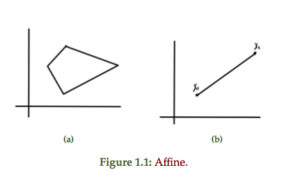For comparison, a couple of non-affine sets are sketched in fig 1.2Definition: Convex set: A set $$C \subseteq \mathbb{R}^n$$ is convex if $$\forall \Bx_1, \Bx_2 \in C$$ and $$\forall \theta \in \mathbb{R}, \theta \in [0,1]$$, the combination

\begin{equation}\label{eqn:convexOptimizationLecture3:700}
\theta \Bx_1 + (1 – \theta) \Bx_2 \in C.
\end{equation}

Definition: Convex combination: A convex combination of $$\Bx_1, \Bx_2, \cdots \Bx_n$$ is

\begin{equation*}
\sum_{i = 1}^n \theta_i \Bx_i,
\end{equation*}

such that $$\forall \theta_i \ge 0$$

\begin{equation*}
\sum_{i = 1}^n \theta_i = 1
\end{equation*}

Definition: Convex hull: Convex hull of a set $$C$$ is a set of all convex combinations of points in $$C$$, denoted

\begin{equation}\label{eqn:convexOptimizationLecture3:720}
\textrm{conv}(C) = \setlr{ \sum_{i=1}^n \theta_i \Bx_i | \Bx_i \in C, \theta_i \ge 0, \sum_{i=1}^n \theta_i = 1 }.
\end{equation}

A non-convex set can be converted into a convex hull by filling in all the combinations of points connecting points in the set, as sketched in fig 1.3.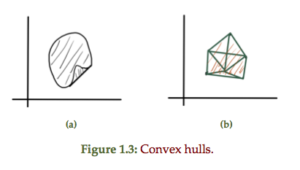Definition: Cone: A set $$C$$ is a cone if $$\forall \Bx \in C$$ and $$\forall \theta \ge 0$$ we have $$\theta \Bx \in C$$.

This scales out if $$\theta > 1$$ and scales in if $$\theta < 1$$.

A convex cone is a cone that is also a convex set. A conic combination is

\begin{equation*}
\sum_{i=1}^n \theta_i \Bx_i, \theta_i \ge 0.
\end{equation*}

A convex and non-convex 2D cone is sketched in fig. 1.4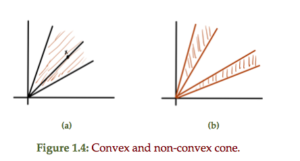A comparison of properties for different set types is tabulated in table 1.1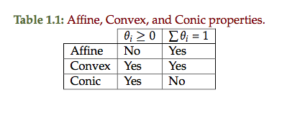## Hyperplanes and half spaces

Definition: Hyperplane: A hyperplane is defined by

\begin{equation*}
\setlr{ \Bx | \Ba^\T \Bx = \Bb, \Ba \ne 0 }.
\end{equation*}

A line and plane are examples of this general construct as sketched in
fig. 1.5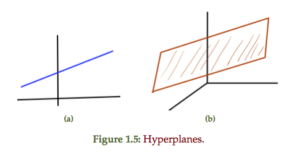An alternate view is possible should one
find any specific $$\Bx_0$$ such that $$\Ba^\T \Bx_0 = \Bb$$

\begin{equation}\label{eqn:convexOptimizationLecture3:740}
\setlr{\Bx | \Ba^\T \Bx = b }
=
\setlr{\Bx | \Ba^\T (\Bx -\Bx_0) = 0 }
\end{equation}

This shows that $$\Bx – \Bx_0 = \Ba^\perp$$ is perpendicular to $$\Ba$$, or

\begin{equation}\label{eqn:convexOptimizationLecture3:780}
\Bx
=
\Bx_0 + \Ba^\perp.
\end{equation}

This is the subspace perpendicular to $$\Ba$$ shifted by $$\Bx_0$$, subject to $$\Ba^\T \Bx_0 = \Bb$$. As a set

\begin{equation}\label{eqn:convexOptimizationLecture3:760}
\Ba^\perp = \setlr{ \Bv | \Ba^\T \Bv = 0 }.
\end{equation}

## Half space

Definition: Half space: The half space is defined as
\begin{equation*}
\setlr{ \Bx | \Ba^\T \Bx = \Bb }
= \setlr{ \Bx | \Ba^\T (\Bx – \Bx_0) \le 0 }.
\end{equation*}

This can also be expressed as $$\setlr{ \Bx | \innerprod{ \Ba }{\Bx – \Bx_0 } \le 0 }$$.